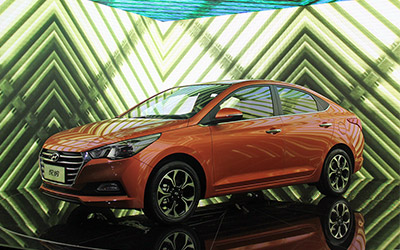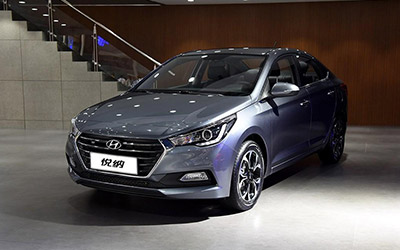# 悦纳本地参考价： 7.28 万元起 厂商指导价：7.28-9.68 万
 车市行情　｜　悦纳 20201.4L CVT精英版TOP降2.10万
 最新年款： 2020款 级　别： 结　构：4门5座三厢轿车 车身颜色： 红色 油　耗：6.8L/100km 排　量：1.4 内饰配色： 红黑 保　修：3年或6万公里 变速箱：6挡手动|CVT无级变速

#### 看过新一代悦纳的配置，才发现合资良心家轿真香！73.3kW(1.4L自然吸气) 自然吸气 官方指导价 本地参考价 车型信息 2020款 1.4L 手动青春版GS 6挡手动变速箱 前置前驱 7.28万暂无 参数　图片　＋对比 2020款 1.4L 手动炫酷版GLS 6挡手动变速箱 前置前驱 7.78万暂无 参数　图片　＋对比 2020款 1.4L CVT炫酷版GLS 无级变速箱 前置前驱 8.78万暂无 参数　图片　＋对比 2020款 1.4L CVT精英版TOP 无级变速箱 前置前驱 9.68万暂无 参数　图片　＋对比

### 绕车实拍 外观 中控 座椅 细节 全部### 悦纳 新闻资讯#### 小资座驾新悦纳 PK卡罗拉#### 新悦纳&全新瑞纳 品质双雄#### 看过新一代悦纳的配置，才发现合资良心家轿真香！#### 特殊时期，车内如何防疫？新一代悦纳已备好攻略#### 新一代悦纳获奖狂撒春节大礼#### 告别薛定谔的火车票 新一代悦纳送你春运攻略#### 新一代悦纳对比威驰谁更全能#### 新一代悦纳加门槛 痛击同级销冠#### 论“靠谱青年”新一代悦纳的自我修养#### 4.9L油耗+颜值无双 新生代智美家轿不容错过》#### 为年轻发声，新一代悦纳如何领航年轻用车潮流？#### 北京现代四款车型等你来购#### 玛莎拉蒂新款Ghibli亮相福布斯精英峰会#### 劲爆来袭 北京现代４款车型惠战金秋#### 北京现代悦纳正式上市 售价7.28-10.58万元

2016年10月18日，北京现代悦纳早在2016成都车展上全球首发，作为瑞纳的换代车型，悦纳无论在颜值、车身尺寸、内饰设计及配置都有了明显升级，...#### 2016成都车展探馆 北京现代悦纳量产版

2016成都国际车展即将在9月2日开启，在此次车展上，北京现代悦纳量产版将正式发布，并计划于10月份上市发售，前方探馆团队在展馆外拍摄到了这...### 猜你喜欢

﻿
• 快速找车
• 选择品牌
• 选择品牌
• A  奥迪
• A  阿斯顿·马丁
• A  阿尔法·罗密欧
• B  宝沃
• B  布加迪
• B  巴博斯
• B  保时捷
• B  宾利
• B  奔驰
• B  宝马
• B  本田
• B  别克
• B  标致
• B  比亚迪
• B  宝骏
• B  北汽制造
• B  北汽新能源
• B  北汽幻速
• B  北汽威旺
• B  北京汽车
• B  奔腾
• B  北汽绅宝
• B  北汽昌河
• C  长安欧尚
• C  长安
• C  长安凯程
• C  长城
• D  大众
• D  道奇
• D  DS
• D  东南
• D  东风风神
• D  东风风行
• D  东风小康
• D  东风风度
• D  东风
• F  福特
• F  丰田
• F  菲亚特
• F  法拉利
• F  福田
• F  福迪
• F  福汽启腾
• G  观致
• G  广汽传祺
• G  广汽吉奥
• G  GMC
• H  红旗
• H  汉腾汽车
• H  哈弗
• H  哈飞
• H  海格
• H  海马
• H  华颂
• H  黄海
• H  华泰
• H  恒天
• J  捷途
• J  几何汽车
• J  捷达
• J  吉利汽车
• J  捷豹
• J  Jeep
• J  江淮
• J  江铃
• J  金杯
• J  九龙
• J  金旅
• K  凯翼
• K  凯迪拉克
• K  克莱斯勒
• K  科尼塞克
• K  卡威
• K  开瑞
• L  路虎
• L  林肯
• L  劳斯莱斯
• L  兰博基尼
• L  雷克萨斯
• L  铃木
• L  领克
• L  雷诺
• L  理念
• L  力帆
• L  莲花汽车
• L  猎豹
• L  路特斯
• L  陆风
• M  马自达
• M  MG
• M  MINI
• M  玛莎拉蒂
• M  摩根
• M  迈凯轮
• N  纳智捷
• O  欧拉
• O  欧宝
• O  讴歌
• O  欧朗
• Q  奇瑞
• Q  起亚
• Q  启辰
• R  日产
• R  荣威
• R  瑞麒
• S  SERES赛力斯
• S  三菱
• S  斯威汽车
• S  萨博
• S  smart
• S  斯柯达
• S  斯巴鲁
• S  思铭
• S  双龙
• S  上汽大通
• S  双环
• T  特斯拉
• T  腾势
• W  蔚来
• W  沃尔沃
• W  WEY
• W  五菱汽车
• W  五十铃
• W  威兹曼
• W  威麟
• X  现代
• X  雪佛兰
• X  星途
• X  雪铁龙
• X  小鹏汽车
• X  西雅特
• Y  一汽
• Y  英菲尼迪
• Y  英致
• Y  依维柯
• Y  野马汽车
• Y  永源
• Z  众泰
• Z  中华
• Z  中兴
• Z  知豆
• 选择车系
• 选择车系
• 车型对比
• 选择品牌
• 选择品牌
• A  奥迪
• A  阿斯顿·马丁
• A  阿尔法·罗密欧
• B  宝沃
• B  布加迪
• B  巴博斯
• B  保时捷
• B  宾利
• B  奔驰
• B  宝马
• B  本田
• B  别克
• B  标致
• B  比亚迪
• B  宝骏
• B  北汽制造
• B  北汽新能源
• B  北汽幻速
• B  北汽威旺
• B  北京汽车
• B  奔腾
• B  北汽绅宝
• B  北汽昌河
• C  长安欧尚
• C  长安
• C  长安凯程
• C  长城
• D  大众
• D  道奇
• D  DS
• D  东南
• D  东风风神
• D  东风风行
• D  东风小康
• D  东风风度
• D  东风
• F  福特
• F  丰田
• F  菲亚特
• F  法拉利
• F  福田
• F  福迪
• F  福汽启腾
• G  观致
• G  广汽传祺
• G  广汽吉奥
• G  GMC
• H  红旗
• H  汉腾汽车
• H  哈弗
• H  哈飞
• H  海格
• H  海马
• H  华颂
• H  黄海
• H  华泰
• H  恒天
• J  捷途
• J  几何汽车
• J  捷达
• J  吉利汽车
• J  捷豹
• J  Jeep
• J  江淮
• J  江铃
• J  金杯
• J  九龙
• J  金旅
• K  凯翼
• K  凯迪拉克
• K  克莱斯勒
• K  科尼塞克
• K  卡威
• K  开瑞
• L  路虎
• L  林肯
• L  劳斯莱斯
• L  兰博基尼
• L  雷克萨斯
• L  铃木
• L  领克
• L  雷诺
• L  理念
• L  力帆
• L  莲花汽车
• L  猎豹
• L  路特斯
• L  陆风
• M  马自达
• M  MG
• M  MINI
• M  玛莎拉蒂
• M  摩根
• M  迈凯轮
• N  纳智捷
• O  欧拉
• O  欧宝
• O  讴歌
• O  欧朗
• Q  奇瑞
• Q  起亚
• Q  启辰
• R  日产
• R  荣威
• R  瑞麒
• S  SERES赛力斯
• S  三菱
• S  斯威汽车
• S  萨博
• S  smart
• S  斯柯达
• S  斯巴鲁
• S  思铭
• S  双龙
• S  上汽大通
• S  双环
• T  特斯拉
• T  腾势
• W  蔚来
• W  沃尔沃
• W  WEY
• W  五菱汽车
• W  五十铃
• W  威兹曼
• W  威麟
• X  现代
• X  雪佛兰
• X  星途
• X  雪铁龙
• X  小鹏汽车
• X  西雅特
• Y  一汽
• Y  英菲尼迪
• Y  英致
• Y  依维柯
• Y  野马汽车
• Y  永源
• Z  众泰
• Z  中华
• Z  中兴
• Z  知豆
• 选择车系
• 选择车系
• 选择车型
• 选择车型
• 意见反馈